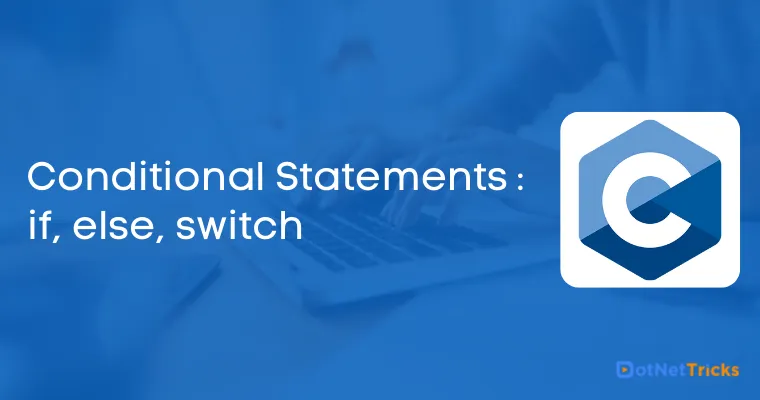# Conditional Statements : if, else, switch

21 Aug 2022
Intermediate
370K Views

Conditional statements help you to make a decision based on certain conditions. These conditions are specified by a set of conditional statements having boolean expressions which are evaluated to a boolean value of true or false.

In our life, we frequently encounter certain situations where we have to make a decision be it your favorite food, movie, hobby, or the color of our shoes. In C programming also, you may encounter similar kinds of situations where you need to make a decision based on the two possibilities that are yes/no or true/false acceptance, we will learn all about the conditional statements in this article.

There are the following types of conditional statements in C.

1. If statement

2. If-Else statement

3. Nested If-else statement

5. Switch statement

## If statement

The single if statement in C language is used to execute the code if a condition is true. It is also called a one-way selection statement. When we use the if condition, we pass the argument and if the argument will be satisfied then the respective code will be executed otherwise nothing can happen.

Below is the if statement followed by the conditional expression.

### Syntax

```if(expression)
{
//code to be executed
}
```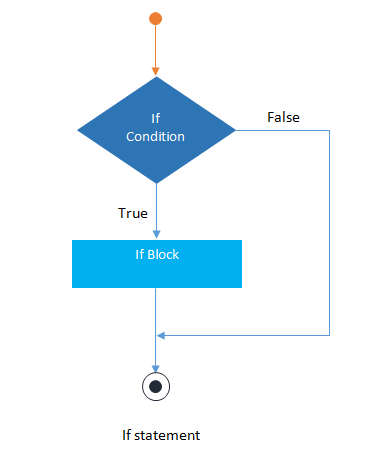### How the "if" statement works

• If the expression is evaluated to nonzero (true) then if block statement(s) are executed.

• If the expression is evaluated to zero (false) then Control passes to the next statement following it.

#### Note

"Expression must be scalar type" i.e evaluated to a single value.

### if Statement Example

```#include<stdio.h>
#include<conio.h>
void main()
{
int num=0;
printf("enter the number");
scanf("%d",&num);
if(n%2==0)
{
printf("%d number in even",num);
}
getch();
}
```

## If-else statement

The if-else statement in C language is used to execute the code if the condition is true or false. It is also called a two-way selection statement.

The single if statement may work pretty well, but if you want to work with multiple variables or the extended conditional parameters, then the if-else statement is the optimum choice. By using the if statement, only one block of the code executes after the condition is true but by using the if-else statement, there are two possible blocks of code where the first block is used for handling the success part and the other one for the failure condition.

### Syntax

```if(expression)
{
//Statements
}
else
{
//Statements
}
```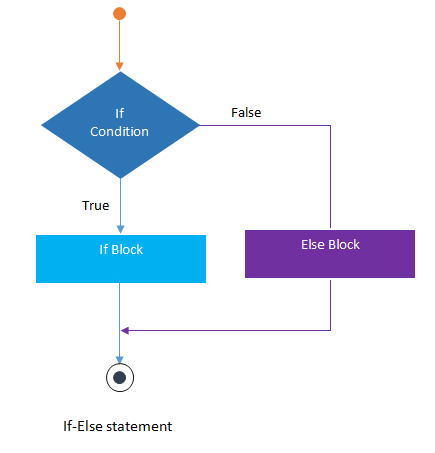### How the "if..else" statement works

• If the expression is evaluated to nonzero (true) then if block statement(s) are executed.

• If the expression is evaluated to zero (false) then else block statement(s) are executed.

### if..else Statement Example

```#include<stdio.h>
#include<conio.h>
void main()
{
int num=0;
printf("enter the number");
scanf("%d",&num);
if(n%2==0)
{
printf("%d number in even", num);
}
else
{
printf("%d number in odd",num);
}
getch();
}
```

## Nested If-else statement

The nested if...else statement is used when a program requires more than one test expression. It is also called a multi-way selection statement. When a series of the decision are involved in a statement, we use the if-else statement in nested form.

Nested if-else statements can be useful when we can have multiple sources of expression and the values and based on the specific value, we need to check nested conditions.

Its recommended for the best practice to avoid using nested if-else statement as it may turn into a conditional bubbling situation, better to use if-else-if or the switch case for the better conditional handling.

### Syntax

```if( expression )
{
if( expression1 )
{
statement-block1;
}
else
{
statement-block 2;
}
}
else
{
statement-block 3;
}
```

### Example

```#include<stdio.h>
#include<conio.h>
void main( )
{
int a,b,c;
clrscr();
scanf("%d%d%d",&a,&b,&c);
if(a>b)
{
if(a>c)
{
printf("a is greatest");
}
else
{
printf("c is greatest");
}
}
else
{
if(b>c)
{
printf("b is greatest");
}
else
{
printf("c is greatest");
}
}
getch();
}
```

The if-else-if statement is used to execute one code from multiple conditions. It is also called a multipath decision statement. It is a chain of if..else statements in which each if statement is associated with an else if statement and the last would be an else statement.

### Syntax

```if(condition1)
{
//statements
}
else if(condition2)
{
//statements
}
else if(condition3)
{
//statements
}
else
{
//statements
}
```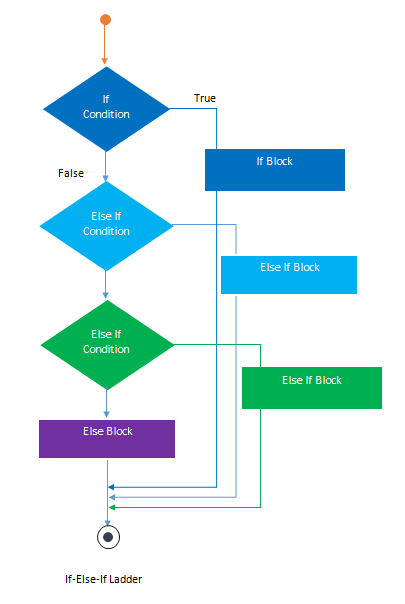```#include<stdio.h>
#include<conio.h>
void main( )
{
int a;
printf("enter a number");
scanf("%d",&a);
if( a%5==0 && a%8==0)
{
printf("divisible by both 5 and 8");
}
else if( a%8==0 )
{
printf("divisible by 8");
}
else if(a%5==0)
{
printf("divisible by 5");
}
else
{
printf("divisible by none");
}
getch();
}
```

## Switch Statement

switch statement acts as a substitute for a long if-else-if ladder that is used to test a list of cases. A switch statement contains one or more case labels that are tested against the switch expression. When the expression match to a case then the associated statements with that case would be executed.

We have seen the way of using conditional statements such as if, if-else. if-else ladder, but the need for an additional way of dealing with conditional statements may seem unnecessary but based on the certain usage, switch case was defined to check for the single condition, and based on the multiple cases, code can be executed.

Below is the basic syntax that shows how to use and implement a switch statement.

### Syntax

```Switch (expression)
{
case value1:
//Statements
break;
case value 2:
//Statements
break;
case value 3:
//Statements
case value n:
//Statements
break;
Default:
//Statements
}

```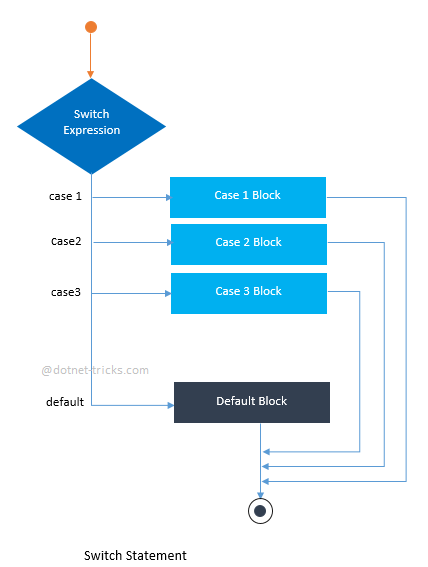### switch statement Example

```#include<stdio.h>
#include<conio.h>
void main( )
{

{
printf("Excellent!");
}
{
printf("Well done");
}
{
printf("You passed");
}
{
printf("Better try again");
}
else
{
printf("You Failed!");
}
}
getch();
}
```
Read More Articles Related to C Programming Language

#### Note

• The switch statement must be an integral type.

• Case labels must be constants.

• Case labels must be unique.

• Case labels must end with a colon.

• The break statement transfers the control out of the switch statement.

• The break statement is optional.

Learn to Crack Your Technical Interview